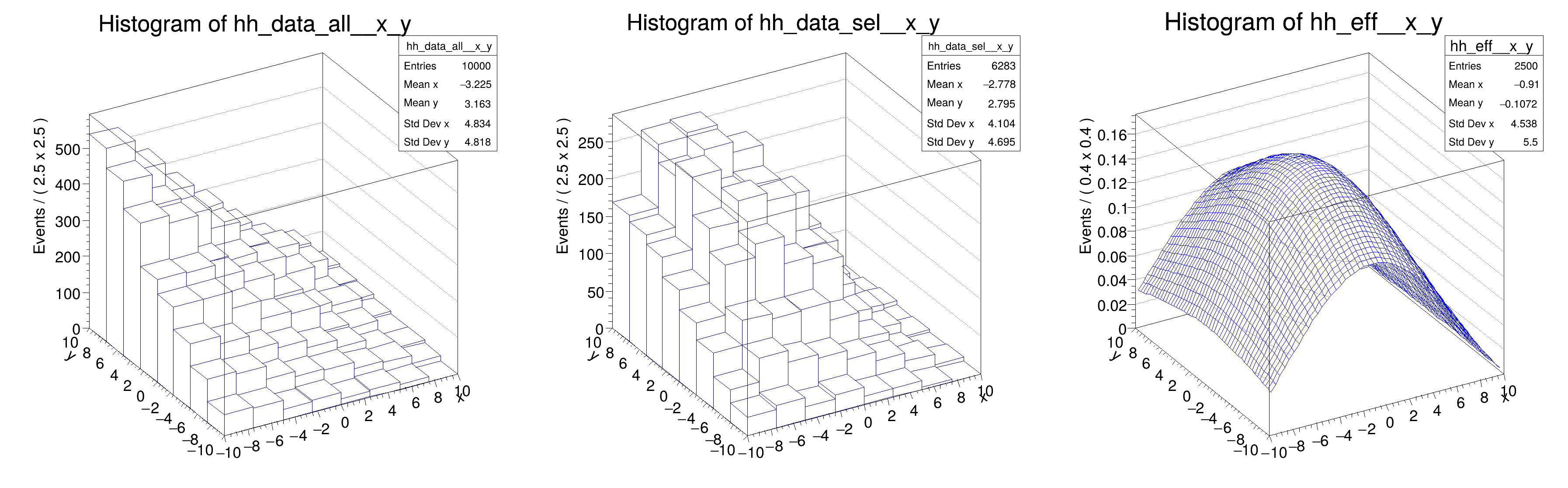ROOT   Reference Guide
Searching...
No Matches
rf702_efficiencyfit_2D.C File Reference

## Detailed DescriptionSpecial pdf's: unbinned maximum likelihood fit of an efficiency eff(x) function to a dataset D(x,cut), cut is a category encoding a selection whose efficiency as function of x should be described by eff(x)#include "RooRealVar.h"
#include "RooDataSet.h"
#include "RooGaussian.h"
#include "RooConstVar.h"
#include "RooCategory.h"
#include "RooEfficiency.h"
#include "RooPolynomial.h"
#include "RooProdPdf.h"
#include "RooFormulaVar.h"
#include "TCanvas.h"
#include "TAxis.h"
#include "TH1.h"
#include "RooPlot.h"
using namespace RooFit;
void rf702_efficiencyfit_2D(bool flat = false)
{
// C o n s t r u c t e f f i c i e n c y f u n c t i o n e ( x , y )
// -----------------------------------------------------------------------
// Declare variables x,mean,sigma with associated name, title, initial value and allowed range
RooRealVar x("x", "x", -10, 10);
RooRealVar y("y", "y", -10, 10);
// Efficiency function eff(x;a,b)
RooRealVar ax("ax", "ay", 0.6, 0, 1);
RooRealVar bx("bx", "by", 5);
RooRealVar cx("cx", "cy", -1, -10, 10);
RooRealVar ay("ay", "ay", 0.2, 0, 1);
RooRealVar by("by", "by", 5);
RooRealVar cy("cy", "cy", -1, -10, 10);
RooFormulaVar effFunc("effFunc", "((1-ax)+ax*cos((x-cx)/bx))*((1-ay)+ay*cos((y-cy)/by))",
RooArgList(ax, bx, cx, x, ay, by, cy, y));
// Acceptance state cut (1 or 0)
RooCategory cut("cut", "cutr", { {"accept", 1}, {"reject", 0} });
// C o n s t r u c t c o n d i t i o n a l e f f i c i e n c y p d f E ( c u t | x , y )
// ---------------------------------------------------------------------------------------------
// Construct efficiency pdf eff(cut|x)
RooEfficiency effPdf("effPdf", "effPdf", effFunc, cut, "accept");
// G e n e r a t e d a t a ( x , y , c u t ) f r o m a t o y m o d e l
// -------------------------------------------------------------------------------
// Construct global shape pdf shape(x) and product model(x,cut) = eff(cut|x)*shape(x)
// (These are _only_ needed to generate some toy MC here to be used later)
RooPolynomial shapePdfX("shapePdfX", "shapePdfX", x, RooConst(flat ? 0 : -0.095));
RooPolynomial shapePdfY("shapePdfY", "shapePdfY", y, RooConst(flat ? 0 : +0.095));
RooProdPdf shapePdf("shapePdf", "shapePdf", RooArgSet(shapePdfX, shapePdfY));
RooProdPdf model("model", "model", shapePdf, Conditional(effPdf, cut));
// Generate some toy data from model
std::unique_ptr<RooDataSet> data{model.generate({x, y, cut}, 10000)};
// F i t c o n d i t i o n a l e f f i c i e n c y p d f t o d a t a
// --------------------------------------------------------------------------
// Fit conditional efficiency pdf to data
// P l o t f i t t e d , d a t a e f f i c i e n c y
// --------------------------------------------------------
// Make 2D histograms of all data, selected data and efficiency function
TH1 *hh_data_all = data->createHistogram("hh_data_all", x, Binning(8), YVar(y, Binning(8)));
TH1 *hh_data_sel = data->createHistogram("hh_data_sel", x, Binning(8), YVar(y, Binning(8)), Cut("cut==cut::accept"));
TH1 *hh_eff = effFunc.createHistogram("hh_eff", x, Binning(50), YVar(y, Binning(50)));
// Some adjustment for good visualization
hh_data_all->SetMinimum(0);
hh_data_sel->SetMinimum(0);
hh_eff->SetMinimum(0);
hh_eff->SetLineColor(kBlue);
// Draw all frames on a canvas
TCanvas *ca = new TCanvas("rf702_efficiency_2D", "rf702_efficiency_2D", 1200, 400);
ca->Divide(3);
ca->cd(1);
hh_data_all->GetZaxis()->SetTitleOffset(1.8);
hh_data_all->Draw("lego");
ca->cd(2);
hh_data_sel->GetZaxis()->SetTitleOffset(1.8);
hh_data_sel->Draw("lego");
ca->cd(3);
hh_eff->GetZaxis()->SetTitleOffset(1.8);
hh_eff->Draw("surf");
return;
}
@ kBlue
Definition Rtypes.h:66
Option_t Option_t TPoint TPoint const char GetTextMagnitude GetFillStyle GetLineColor GetLineWidth GetMarkerStyle GetTextAlign GetTextColor GetTextSize void data
RooArgList is a container object that can hold multiple RooAbsArg objects.
Definition RooArgList.h:22
RooArgSet is a container object that can hold multiple RooAbsArg objects.
Definition RooArgSet.h:55
RooCategory is an object to represent discrete states.
Definition RooCategory.h:28
RooEfficiency is a PDF helper class to fit efficiencies parameterized by a supplied function F.
A RooFormulaVar is a generic implementation of a real-valued object, which takes a RooArgList of serv...
RooPolynomial implements a polynomial p.d.f of the form.
RooProdPdf is an efficient implementation of a product of PDFs of the form.
Definition RooProdPdf.h:33
RooRealVar represents a variable that can be changed from the outside.
Definition RooRealVar.h:37
virtual void SetTitleOffset(Float_t offset=1)
Set distance between the axis and the axis title.
Definition TAttAxis.cxx:298
virtual void SetLineColor(Color_t lcolor)
Set the line color.
Definition TAttLine.h:40
virtual void SetLeftMargin(Float_t leftmargin)
The Canvas class.
Definition TCanvas.h:23
Definition TCanvas.cxx:716
TH1 is the base class of all histogram classes in ROOT.
Definition TH1.h:58
TAxis * GetZaxis()
Definition TH1.h:324
void Draw(Option_t *option="") override
Draw this histogram with options.
Definition TH1.cxx:3063
virtual void SetMinimum(Double_t minimum=-1111)
Definition TH1.h:401
void Divide(Int_t nx=1, Int_t ny=1, Float_t xmargin=0.01, Float_t ymargin=0.01, Int_t color=0) override
RooCmdArg YVar(const RooAbsRealLValue &var, const RooCmdArg &arg={})
RooCmdArg Conditional(const RooArgSet &pdfSet, const RooArgSet &depSet, bool depsAreCond=false)
RooConstVar & RooConst(double val)
RooCmdArg PrintLevel(Int_t code)
RooCmdArg ConditionalObservables(Args_t &&... argsOrArgSet)
Create a RooCmdArg to declare conditional observables.
RooCmdArg Binning(const RooAbsBinning &binning)
RooCmdArg Cut(const char *cutSpec)
Double_t y[n]
Definition legend1.C:17
Double_t x[n]
Definition legend1.C:17
The namespace RooFit contains mostly switches that change the behaviour of functions of PDFs (or othe...
[#0] WARNING:Generation -- RooAcceptReject::ctor(effPdf_Int[]_Norm[cut]) WARNING: performing accept/reject sampling on a p.d.f in 2 dimensions without prior knowledge on maximum value of p.d.f. Determining maximum value by taking 200000 trial samples. If p.d.f contains sharp peaks smaller than average distance between trial sampling points these may be missed and p.d.f. may be sampled incorrectly.
[#0] WARNING:Generation -- RooAcceptReject::ctor(effPdf_Int[]_Norm[cut]): WARNING: 200000 trial samples requested by p.d.f for 2-dimensional accept/reject sampling, this may take some time
[#1] INFO:Minimization -- RooAbsMinimizerFcn::setOptimizeConst: activating const optimization
[#1] INFO:Minimization -- RooAbsMinimizerFcn::setOptimizeConst: deactivating const optimization
Date
February 2018

Definition in file rf702_efficiencyfit_2D.C.